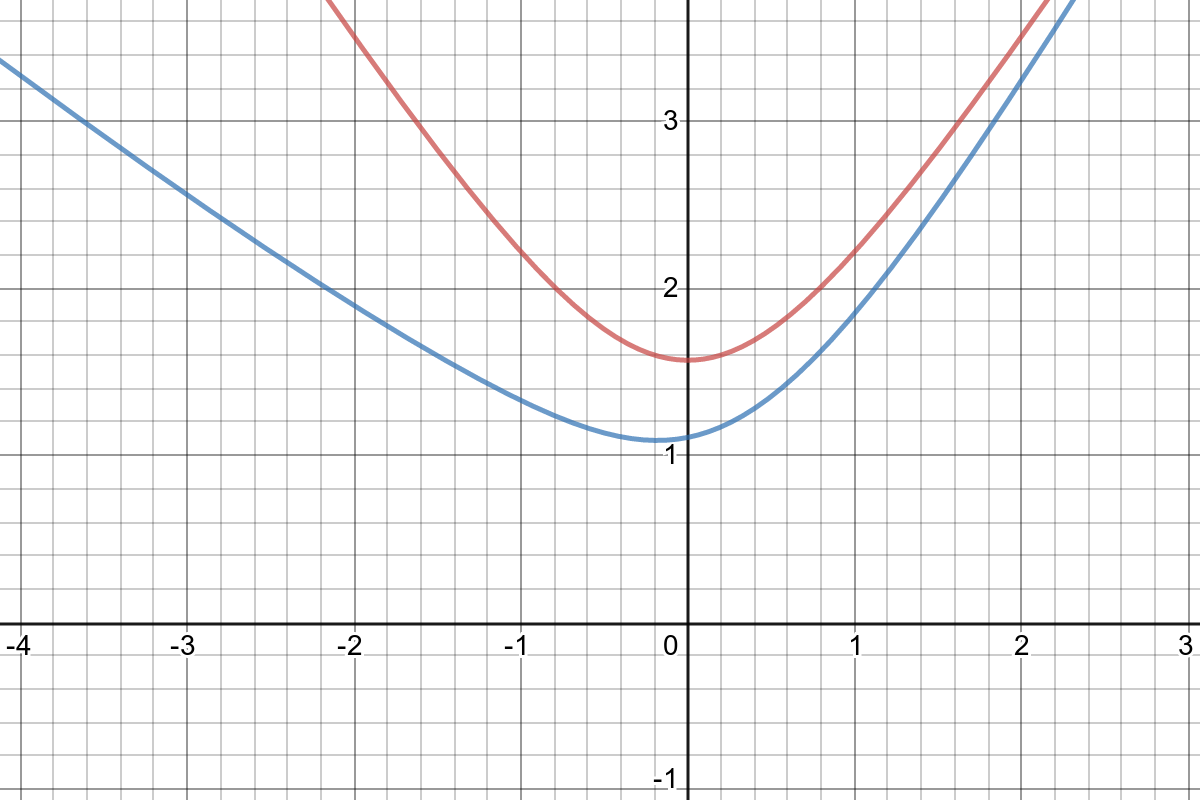### C - Chinese curves

##### Languages: C, C++, Java, Python, ... (details)

You are in a world with curves of the form $f(x) = \arctan(e ^ x + a) \sqrt{b \cdot x^2 + c}$, where $a$, $b$ and $c$ are integers. In this world, there are two types of queries:
• Given three integers $a$, $b$ and $c$, create a new curve with parameters $a$, $b$ and $c$.
• Given an integer $p$, determine the minimum value of $f(p)$ among all curves.

The following image corresponds to the sample test case, where the blue curve is the last curve added.Here is an explanation of the sample input:

1. From the input, mask $m$ is intially set to $0$.
2. Query 1 creates a curve with parameter $a = 1000$, $b = 1$, $c = 1$ (since mask $m = 0$).
3. Query 2 asks for the minimum value of $f(1)$ among all curves.  Since there is only one curve, answer is simply $\arctan(e ^ 1 + 1000) \sqrt{1^2 + 1}$, which is about $2.22$.
4. After answering query 2, mask $m$ becomes $\lfloor 2.22 \rfloor \oplus 0 = 2$.
5. Query 3 creates a curve with parameters $a$, $b$, and $c$ equal to $3 \oplus 2 = 1$.
6. As $p = 3 \oplus 2 = 1$, query 4 asks for the minimum value of $f(1)$ among the two existing curves.  From the image, we can see that the last added curve yields a minimum value of  about $1.85$.
7. After answering query 4, mask $m$ is updated to $\lfloor 1.85 \rfloor \oplus 2 = 3$.
8. The last query asks to find the minimum value of $f(41 \oplus 3) = f(42)$ among the two curves.

#### Input

In the first line, there are two integers $q$ and $m$, where $q$ is the number of queries to follow $(1 \le q \le 10 ^ 5)$ and $m$ is a mask $(0 \le m \le 10 ^ 5)$. Each of the following $q$ lines contains a query using one of these two formats:
• $1$ $a$ $b$ $c$: if the query is of the first type, where $0 \le b \le 10 ^ 6$, $0 \le a, c \le 10 ^{18}$.
• $2$ $p$: if the query is of the second type, where $0 \le p \le 10 ^ 6$.
In the input, the values of $a$, $b$, $c$ and $p$ are not given directly. Instead, you should restore them from the inputs $a'$, $b'$, $c'$ and $p'$ by computing $a = a' \oplus m$, $b = b' \oplus m$, $c = c' \oplus m$, and $p = p' \oplus m$, where $m$ is the current value of mask $m$ and $\oplus$ is the bitwise xor operator (available as the ^ symbol in many programming languages).

After each query of type 2, the mask $m$ changes to $\lfloor s \rfloor \oplus m$, where $s$ is the answer to the query, $\oplus$ is the bitwise xor operator, and $\lfloor s \rfloor$ is the floor function that yields the integer part of number $s$.

It is guaranteed that the first query is of type 1.

#### Output

For each query of type 2, output a line with the required answer, rounding to two digits of precision after the decimal point.

#### Sample test(s)

Input
5 0 1 1000 1 1 2 1 1 3 3 3 2 3 2 41
Output
2.22 1.85 65.99